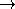## CGAL::Extremal_polygon_perimeter_traits_2<K>advanced### Definition

The class Extremal_polygon_perimeter_traits_2 provides the types and operations needed to compute a maximum perimeter k-gon Pk that can be inscribed into a given convex polygon P using the function extremal_polygon_2.

### Requirements

The template parameter K is a model for Kernel.

### Is Model for the Concept

ExtremalPolygonTraits_2

### Types

 Extremal_polygon_perimeter_traits_2::FT typedef to K::FT. Extremal_polygon_perimeter_traits_2::Point_2 typedef to K::Point_2. Extremal_polygon_perimeter_traits_2::Less_xy_2 typedef to K::Less_xy_2. Extremal_polygon_perimeter_traits_2::Orientation_2 typedef to K::Orientation_2. Extremal_polygon_perimeter_traits_2::Operation AdaptableBinaryFunction class op: Point_2  × Point_2FT. For a fixed Point_2 root, op(p,q) returns d(r,p) + d(p,q) - d(r,q) where d denotes the Euclidean distance.

### Operations

int t.min_k () const returns 2.

FT t.init ( const Point_2& p, const Point_2& q) const
returns twice the Euclidean distance between p and q.

Operation t.operation ( const Point_2& p) const
returns Operation where p is the fixed root point.

template < class RandomAccessIterator, class OutputIterator >
OutputIterator
 t.compute_min_k_gon ( RandomAccessIterator points_begin, RandomAccessIterator points_end, FT& max_area, OutputIterator o)
const
writes the pair (points_begin, p) where p is drawn from [points_begin, points_end) such that the Euclidean distance between both points is maximized (maximum perimeter 2-gon rooted at points_begin) to o and returns the past-the-end iterator for that sequence (== o + 2).

Less_xy_2 t.less_xy_2_object ()
Orientation_2 t.orientation_2_object ()advanced